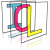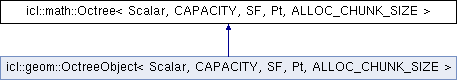Image Component Library (ICL)
icl::math::Octree< Scalar, CAPACITY, SF, Pt, ALLOC_CHUNK_SIZE > Class Template Reference

Generic Octree Implementation. More...

`#include <Octree.h>`

Inheritance diagram for icl::math::Octree< Scalar, CAPACITY, SF, Pt, ALLOC_CHUNK_SIZE >:## Classes

struct  AABB
internally used axis-aligned bounding box More...

struct  Allocator
Inernally used block allocator. More...

struct  Node
Internally used node structure. More...

## Public Member Functions

Octree (const Scalar &minX, const Scalar &minY, const Scalar &minZ, const Scalar &width, const Scalar &height, const Scalar &depth)
creates a QuadTree for the given 2D rectangle More...

Octree (const Scalar &min, const Scalar &len)
creates a QuadTree for the given 2D rectangle More...

~Octree ()
destructor More...

const AABBgetRootAABB () const
returs the Octree's top-level bounding box More...

Pt nn_approx (const Pt &p) const
returns an approximated nearst neighbour More...

Pt nn (const Pt &pIn) const
finds the nearest neighbor to the given node More...

template<class OtherVectorType >
void insert (const OtherVectorType &pIn)
inserts a node into the QuadTree More...

template<class ForwardIterator >
void assign (ForwardIterator begin, ForwardIterator end)
utilty method to assign new data More...

std::vector< Pt > query (const Scalar &minX, const Scalar &minY, const Scalar &minZ, const Scalar &width, const Scalar &height, const Scalar &depth) const
returns all contained points within the given rectangle More...

std::vector< Pt > queryAll () const
returns all contained points More...

void clear ()
removes all contained points and nodes More...

int size () const
number of elments inserted More...

## Protected Member Functions

const Pt & nn_approx_internal (const Pt &p, double &currMinDist, const Pt *&currNN) const
internal utility method that is used to find an approximated nearest neighbour More...

## Static Protected Member Functions

static Pt scale_up (const Pt &p)

static Pt scale_down (const Pt &p)

static Pt scale_down_1 (const Pt &p)

## Protected Attributes

Noderoot
root node pointer More...

Allocator alloc
memory allocator for all children except for the root node More...

int num
internal counter for the number of contained points More...

## Detailed Description

### template<class Scalar, int CAPACITY = 16, int SF = 1, class Pt = FixedColVector<Scalar,4>, int ALLOC_CHUNK_SIZE = 1024> class icl::math::Octree< Scalar, CAPACITY, SF, Pt, ALLOC_CHUNK_SIZE >

Generic Octree Implementation.

The Octree implementation is a simple 3D-generalization of the QuadTree class template.

# Benchmarks:

Even though, we do not have any reliable results, it might be possible, that the octree is much faster then the pcl-octree!

## ◆ Octree() [1/2]

template<class Scalar, int CAPACITY = 16, int SF = 1, class Pt = FixedColVector<Scalar,4>, int ALLOC_CHUNK_SIZE = 1024>
 icl::math::Octree< Scalar, CAPACITY, SF, Pt, ALLOC_CHUNK_SIZE >::Octree ( const Scalar & minX, const Scalar & minY, const Scalar & minZ, const Scalar & width, const Scalar & height, const Scalar & depth )
inline

creates a QuadTree for the given 2D rectangle

## ◆ Octree() [2/2]

template<class Scalar, int CAPACITY = 16, int SF = 1, class Pt = FixedColVector<Scalar,4>, int ALLOC_CHUNK_SIZE = 1024>
 icl::math::Octree< Scalar, CAPACITY, SF, Pt, ALLOC_CHUNK_SIZE >::Octree ( const Scalar & min, const Scalar & len )
inline

creates a QuadTree for the given 2D rectangle

## ◆ ~Octree()

template<class Scalar, int CAPACITY = 16, int SF = 1, class Pt = FixedColVector<Scalar,4>, int ALLOC_CHUNK_SIZE = 1024>
 icl::math::Octree< Scalar, CAPACITY, SF, Pt, ALLOC_CHUNK_SIZE >::~Octree ( )
inline

destructor

Deletes the root node only, all other nodes are deleted by the allocator

## ◆ assign()

template<class Scalar, int CAPACITY = 16, int SF = 1, class Pt = FixedColVector<Scalar,4>, int ALLOC_CHUNK_SIZE = 1024>
template<class ForwardIterator >
 void icl::math::Octree< Scalar, CAPACITY, SF, Pt, ALLOC_CHUNK_SIZE >::assign ( ForwardIterator begin, ForwardIterator end )
inline

utilty method to assign new data

Internally, this is implemented using clear() followed by a for-loop based insertion of all the points.

## ◆ clear()

template<class Scalar, int CAPACITY = 16, int SF = 1, class Pt = FixedColVector<Scalar,4>, int ALLOC_CHUNK_SIZE = 1024>
 void icl::math::Octree< Scalar, CAPACITY, SF, Pt, ALLOC_CHUNK_SIZE >::clear ( )
inline

removes all contained points and nodes

The allocator will free all memory except for the first CHUNK

## ◆ getRootAABB()

template<class Scalar, int CAPACITY = 16, int SF = 1, class Pt = FixedColVector<Scalar,4>, int ALLOC_CHUNK_SIZE = 1024>
 const AABB& icl::math::Octree< Scalar, CAPACITY, SF, Pt, ALLOC_CHUNK_SIZE >::getRootAABB ( ) const
inline

returs the Octree's top-level bounding box

## ◆ insert()

template<class Scalar, int CAPACITY = 16, int SF = 1, class Pt = FixedColVector<Scalar,4>, int ALLOC_CHUNK_SIZE = 1024>
template<class OtherVectorType >
 void icl::math::Octree< Scalar, CAPACITY, SF, Pt, ALLOC_CHUNK_SIZE >::insert ( const OtherVectorType & pIn )
inline

inserts a node into the QuadTree

This method is also implemented in an iterative fashion for performance issues. 'insert' automatically uses the internal allocator if new nodes are needed.

## ◆ nn()

template<class Scalar, int CAPACITY = 16, int SF = 1, class Pt = FixedColVector<Scalar,4>, int ALLOC_CHUNK_SIZE = 1024>
 Pt icl::math::Octree< Scalar, CAPACITY, SF, Pt, ALLOC_CHUNK_SIZE >::nn ( const Pt & pIn ) const
inline

finds the nearest neighbor to the given node

The implementation of this method explicitly avoids recursion by using a run-time stack. This leads to a 4x speed factor in comparison to the recursive implementaiton of this function.

As an extra accelleration, the method initializes it's frist nearest neighbor guess using the nn_approx method, which gives an approximate speed up of factor two to four.

As a 2nd accelleration heuristic, all CAPACITY nodes' distances are are first calculated and compared in a squared version, which can be computed without an expensive square-root operation. However, once the closest point within a single node is found, its real euclidian minimum distance is computed and stored for further bounding box checks.

If no neighbour could be found, an exception is thown. This should actually only happen when nn is called on an empty QuadTree

## ◆ nn_approx()

template<class Scalar, int CAPACITY = 16, int SF = 1, class Pt = FixedColVector<Scalar,4>, int ALLOC_CHUNK_SIZE = 1024>
 Pt icl::math::Octree< Scalar, CAPACITY, SF, Pt, ALLOC_CHUNK_SIZE >::nn_approx ( const Pt & p ) const
inline

returns an approximated nearst neighbour

While the real nearst neighbour must not neccessarily be in the cell that would theoretically contain p, The approximated one is always assumed to be in that bottom layer cell. If, by chance, the optimal leaf node does not contain any points (because it was just created as empty leaf), the leaf's parent node, which must actually contain CAPACITY points, is used instead. The approximate nearest neighbour search can easily be 5 times as fast as the real nearest neighbor search. The result quality depends on the number of contained points, and on the QuadTree's template parameters

## ◆ nn_approx_internal()

template<class Scalar, int CAPACITY = 16, int SF = 1, class Pt = FixedColVector<Scalar,4>, int ALLOC_CHUNK_SIZE = 1024>
 const Pt& icl::math::Octree< Scalar, CAPACITY, SF, Pt, ALLOC_CHUNK_SIZE >::nn_approx_internal ( const Pt & p, double & currMinDist, const Pt *& currNN ) const
inlineprotected

internal utility method that is used to find an approximated nearest neighbour

## ◆ query()

template<class Scalar, int CAPACITY = 16, int SF = 1, class Pt = FixedColVector<Scalar,4>, int ALLOC_CHUNK_SIZE = 1024>
 std::vector icl::math::Octree< Scalar, CAPACITY, SF, Pt, ALLOC_CHUNK_SIZE >::query ( const Scalar & minX, const Scalar & minY, const Scalar & minZ, const Scalar & width, const Scalar & height, const Scalar & depth ) const
inline

returns all contained points within the given rectangle

## ◆ queryAll()

template<class Scalar, int CAPACITY = 16, int SF = 1, class Pt = FixedColVector<Scalar,4>, int ALLOC_CHUNK_SIZE = 1024>
 std::vector icl::math::Octree< Scalar, CAPACITY, SF, Pt, ALLOC_CHUNK_SIZE >::queryAll ( ) const
inline

returns all contained points

## ◆ scale_down()

template<class Scalar, int CAPACITY = 16, int SF = 1, class Pt = FixedColVector<Scalar,4>, int ALLOC_CHUNK_SIZE = 1024>
 static Pt icl::math::Octree< Scalar, CAPACITY, SF, Pt, ALLOC_CHUNK_SIZE >::scale_down ( const Pt & p )
inlinestaticprotected

## ◆ scale_down_1()

template<class Scalar, int CAPACITY = 16, int SF = 1, class Pt = FixedColVector<Scalar,4>, int ALLOC_CHUNK_SIZE = 1024>
 static Pt icl::math::Octree< Scalar, CAPACITY, SF, Pt, ALLOC_CHUNK_SIZE >::scale_down_1 ( const Pt & p )
inlinestaticprotected

## ◆ scale_up()

template<class Scalar, int CAPACITY = 16, int SF = 1, class Pt = FixedColVector<Scalar,4>, int ALLOC_CHUNK_SIZE = 1024>
 static Pt icl::math::Octree< Scalar, CAPACITY, SF, Pt, ALLOC_CHUNK_SIZE >::scale_up ( const Pt & p )
inlinestaticprotected

## ◆ size()

template<class Scalar, int CAPACITY = 16, int SF = 1, class Pt = FixedColVector<Scalar,4>, int ALLOC_CHUNK_SIZE = 1024>
 int icl::math::Octree< Scalar, CAPACITY, SF, Pt, ALLOC_CHUNK_SIZE >::size ( ) const
inline

number of elments inserted

## ◆ alloc

template<class Scalar, int CAPACITY = 16, int SF = 1, class Pt = FixedColVector<Scalar,4>, int ALLOC_CHUNK_SIZE = 1024>
 Allocator icl::math::Octree< Scalar, CAPACITY, SF, Pt, ALLOC_CHUNK_SIZE >::alloc
protected

memory allocator for all children except for the root node

## ◆ num

template<class Scalar, int CAPACITY = 16, int SF = 1, class Pt = FixedColVector<Scalar,4>, int ALLOC_CHUNK_SIZE = 1024>
 int icl::math::Octree< Scalar, CAPACITY, SF, Pt, ALLOC_CHUNK_SIZE >::num
protected

internal counter for the number of contained points

## ◆ root

template<class Scalar, int CAPACITY = 16, int SF = 1, class Pt = FixedColVector<Scalar,4>, int ALLOC_CHUNK_SIZE = 1024>
 Node* icl::math::Octree< Scalar, CAPACITY, SF, Pt, ALLOC_CHUNK_SIZE >::root
protected

root node pointer

The documentation for this class was generated from the following file:
• /Users/alneuman/vm/icl/ICLMath/src/ICLMath/Octree.h